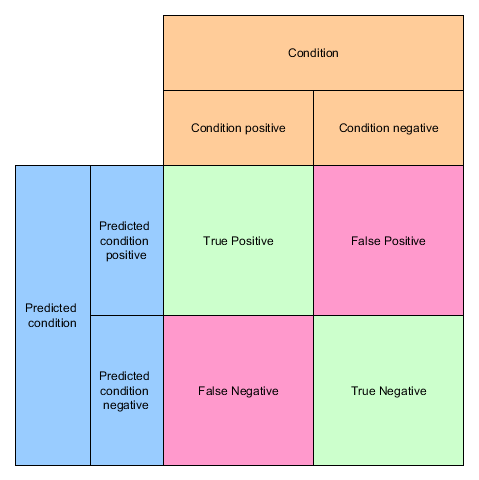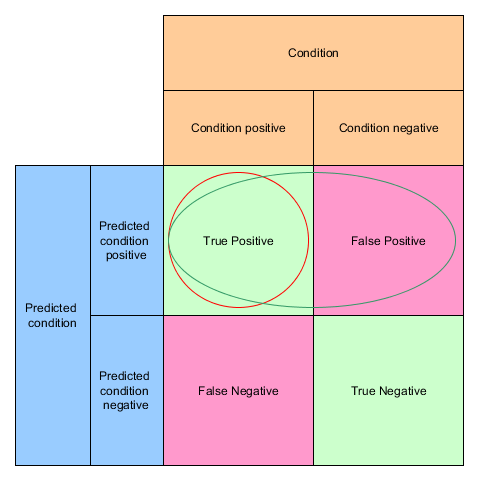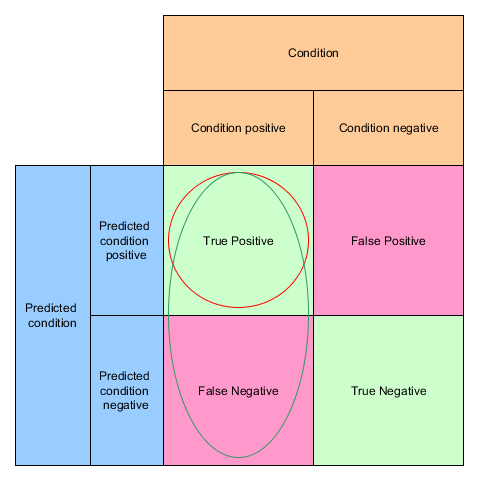In the realms of Data Science you’ll encounter sooner or the later the terms “Precision” and “Recall”. But what do they mean?## Clarification

Living together with little kids You very often run into classification issues:

My daughter really likes dogs, so seeing a dog is something positive. When she sees a normal dog e.g. a Labrador and proclaims: “Look, there is a dog!”

That’s a True Positive (TP)

If she now sees a fat cat and proclaims: “Look at the dog!” we call it a False Positive (FP), because her assumption of a positive outcome (a dog!) was false. A false positive is also called a Type 1 error

If I point at a small dog e.g. a Chihuahua and say “Look at the dog!” and she cries: “This is not a dog!” but indeed it is one, we call that a False negatives (FN) A false negativeis also called a Type 2 error

And last but not least, if I show her a bird and we agree on the bird not being a dog we have a True Negative (TN)

This neat little matrix shows all of them in context:## Precision and Recall

If I show my daughter twenty pictures of cats and dogs (8 cat pictures and 12 dog pictures) and she identifies 10 as dogs but out of ten dogs there are actually 2 cats her precision is 8 / (8+2) = 4/5 or 80%.

Precision = TP / (TP + FP)Knowing that there are actually 12 dog pictures and she misses 4 (false negatives) her recall is 8 / (8+4) = 2/3 or roughly 67%

Recall = TP / (TP + FN)Which measure is more important?

It depends:

If you’re a dog lover it is better to have a high precision, when you are afraid of dogs say to avoid dogs, a higher recall is better 🙂

### Different terms

Precision is also called Positive Predictive Value (PPV)

Recall often is also called

• True positive rate
• Sensitivity
• Probability of detection

## Accuracy

ACC = (TP + TN) / (TP + FP + TN + FN)### F1-Score

You can combine Precision and Recall to a measure called F1-Score. It is the harmonic mean of precision and recall

F1 = 2 / (1/Precision + 1/Recall)

### Scikit-Learn

scikit-learn being a one-stop-shop for data scientists does of course offer functions for calculating precision and recall:

```from sklearn.metrics import precision_score

y_true = ["dog", "dog", "not-a-dog", "not-a-dog", "dog", "dog"]
y_pred = ["dog", "not-a-dog", "dog", "not-a-dog", "dog", "not-a-dog"]

print(precision_score(y_true, y_predicted , pos_label="dog"))```

Let’s assume we trained a binary classifier which can tell us “dog” or “not-a-dog”

In this example the precision is 0.666 or ~67% because in two third of the cases the algorithm was right when it predicted a dog

```from sklearn.metrics import recall_score

print(recall_score(y_true, y_pred, pos_label="dog"))```

The recall was just 0.5 or 50% because out of 4 dogs it just identified 2  correctly as dogs.

```from sklearn.metrics import accuracy_score

print(accuracy_score(y_true, y_pred))```

The accuracy was also just 50% because out of 6 items it made only 3 correct predictions.

```from sklearn.metrics import f1_score

print(f1_score(y_true, y_pred, pos_label="dog"))```

The F1 score is 0.57 – just between 0.5 and 0.666.

What other scores do you encounter? – stay tuned for the next episode 🙂